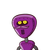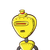# 7. Find the area of a right triangle whose base is 1.2 m and hypotenuse 3.7 m.​

7. Find the area of a right triangle whose base is 1.2 m and hypotenuse 3.7 m.

### 2 thoughts on “<br />7. Find the area of a right triangle whose base is 1.2 m and hypotenuse 3.7 m.<br />​”

1.• Area of a right triangle is 2.1 m².

Step-by-step explanation:

Given that:

• Base of a right triangle = 1.2 m
• Hypotenuse of a right triangle = 3.7 m

To Find:

• Area of a right triangle.

First finding the height of a right triangle:

By pythagoras theorem.

⟶ H² = P² + B²

⟶ (3.7)² = P² + (1.2)²

⟶ 13.69 = P² + 1.44

⟶ P² = 13.69 – 1.44

⟶ P² = 12.25

⟶ P = √12.25

⟶ P = 3.5

∴ Perpendicular/Height of a right triangle = 3.5 m

Finding the area of a right triangle:

⇒ Area = (B × P)/2

⇒ Area = (1.2 × 3.5)/2

⇒ Area = 2.1

∴ Area of a right triangle = 2.1 m²

Some abbreviations used are:

• H = Hypotenuse
• P = Perpendicular/Height
• B = Base
2.we know that:

Area of right angle triangle = 1/2 * Base * perpendicular height

And

According to Pythagoras theorem

(Hypotenuse)²  = (Base)² + (perpendicular)²

Here,

Base = 1.2 m

Hypotenuse = 3.7 m

=> Perpendicular = √(H² – B²)

= √ (3.7² – 1.2²)

= √ (13.69 – 1.44)

= √ 12.25

= 3.5 m

Now,

=> Area  = 1/2 * B * P

= 1/2 * 1.2 * 3.5

= 1/2 * 4.2